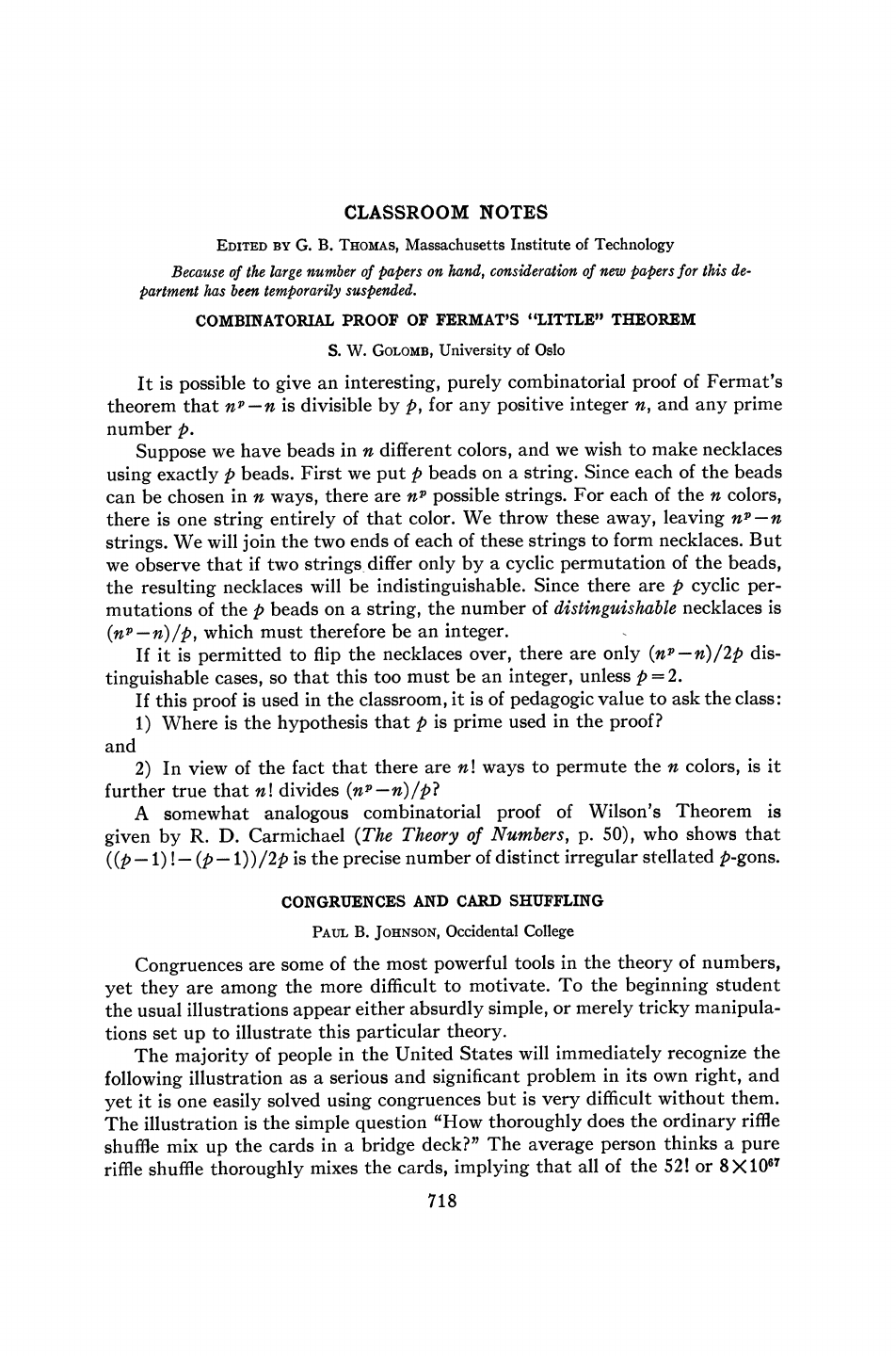Pierre de Fermat first stated what would become his ”Little" Theore...
For simplicity, let's assume we only have 2 colors {R,G} ($n=2$) an...
Note that the number of cyclic permutations of a string of size $p$...
Would a learner appreciate double factorials?Hi Tony, there aren't any double factorials in the proof. Note that the number of cyclic permutations of a string of size $p$ that result in the same necklace is only equal to $p$ if $p$ is prime. If the size of the string is not a prime it might be possible to divide the string into other sub-strings of smaller size and so we need to take into account other permutations between the sub-strings. For instance, for p=4 the string RGRG has only one cyclic permutation that results in the same necklace (RGRG $\equiv$ GRGR). Would a learner appreciate double factorials? From a purely beginner approach to the Pigeon-Hole Problem Add cases. Counting Principle of place value (order, distinguishable) The Pigeon-Hole Problem is dependent on the modular congruence, right? Not one nest left unfilled. Thanks, could one tell me how to remove the comment? For simplicity, let's assume we only have 2 colors {R,G} ($n=2$) and the necklaces have 3 beads ($p=3$). In that case, there are $2^{3}=8$ possible strings of beads. RRR, GRR, RGR, RRG, RGG, GRG, GGR, GGG Now we bring the ends of each string together to create necklaces. We consider two strings as the same necklace if we can rotate one string to obtain the second string. We can observe that for each non-constant string there are precisely p=3 strings (3 cyclic permutations of the beads) that determine the same necklace. For instance, GRR $\equiv$ RGR $\equiv$ RRG , which is the same necklace. As a consequence, the number of distinguishable necklaces is the total number of strings $2^3$ minus the strings made of the same bead (RRR,GGG) divided by the number of permutations that result in equivalent necklaces : $\frac{2^{3}-2}{3}=2$. Generalizing the result for an arbitrary positive integer $n$ and prime $p$ we get $\frac{n^{p}-n}{p}=k$. Since $k$ is just the number of distinguishable necklaces it must therefore be an integer. Pierre de Fermat first stated what would become his ”Little" Theorem in a letter dated October 18, 1640, to his friend [Frénicle de Bessy](https://en.wikipedia.org/wiki/Bernard_Fr%C3%A9nicle_de_Bessy). Fermat did not include a proof for fear the proof would be too long. The first proof of this theorem was published more than fifty years later by Leonhard Euler, in 1736. In this paper S.W.Golomb proves Fermat's "Little" Theorem using only some basic combinatorial machinery.